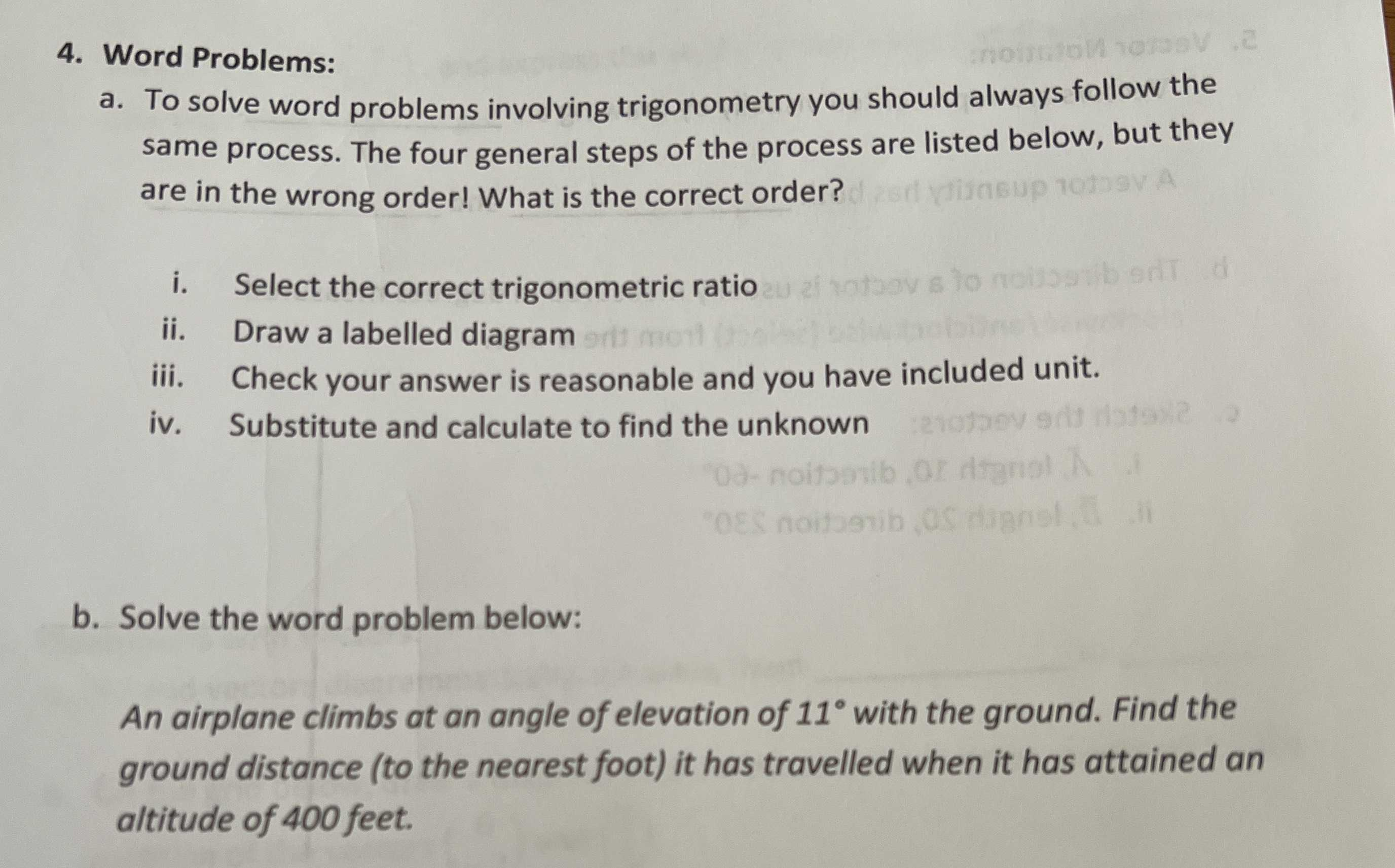### ¿Todavía tienes preguntas de matemáticas?

Pregunte a nuestros tutores expertos
Algebra
Pregunta4. Word Problems:

a. To solve word problems involving trigonometry you should always follow the same process. The four general steps of the process are listed below, but they are in the wrong order! What is the correct order?

i. Select the correct trigonometric ratio

ii. Draw a labelled diagram

iv. Substitute and calculate to find the unknown

b. Solve the word problem below:

An airplane climbs at an angle of elevation of $$11 ^ { \circ }$$ with the ground. Find the ground distance (to the nearest foot) it has travelled when it has attained an altitude of $$400$$ feet.

b.$$\frac{400}{tan11° }$$=2057.8feet